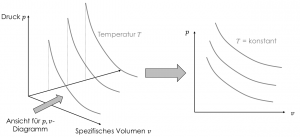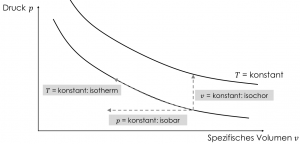# What are isochores

## Change of state

Many complicated relationships can be easily represented in pictures and diagrams. In thermodynamics, the state variables and their changes in systems can be represented in so-called \$ p, v \$ diagrams. The state of a system can be clearly represented with pressure, temperature and specific volume.

Thermodynamics simply explained! Our book on the online learning portal

19,99€

State of a system Z = f (v, p, T)

The equation shows that the state of a system is a three-dimensional function with the parameters \$ p, v \$ and \$ T \$. This is confusing and not necessary for our purposes in Thermodynamics 1. The trick is to display curves of constant temperature from the three-dimensional diagram one above the other for the \$ p, v \$ diagram.Curves of constant temperature are called isotherms (from the Greek word "isos"). The isotherms stand for the ratio of the change in pressure and specific volume at constant temperature. For the example in the figure we see that for a temperature the pressure decreases with increasing specific volume. We will continuously expand our understanding of the behavior of substances step by step.

Starting from a fixed state point, we can change two state variables at a time, while we hold the third state variable. Depending on which of the three state variables \$ p, v \$ or \$ T \$ is left constant, we can define three state changes and represent them in the \$ p, v \$ diagram.The three state changes are:

• Isothermal change of state:Change of pressure and specific volume at constant temperature
• I.Similar change of state:Change of temperature and specific volume at constant pressure
• Isochore change of state:Change of temperature and pressure with constant specific volume

That sounds very theoretical at first, so we'll close the section with an example.

#### Changes of state in a piston pump

In a container of mass \$ m \$ and volume \$ V \$ (from which a specific volume \$ v \$ results) there is a pressure \$ p \$ at a temperature \$ T \$. The container is now heated. Which change of state is it?

1. The temperature rises, but the air in the pump cannot expand due to the supply of heat, so the volume remains constant. It is an isochoric change of state with increasing pressure.

The container has a sliding piston at the top as a lid. Heat continues to be supplied. Which change of state is it now?

2. The addition of heat increases the temperature of the air. The piston rises, which changes the volume, so the specific volume also changes. The change of state is isobaric. As soon as we can calculate changes in state, we will recalculate and substantiate this plausibility consideration.

The container is then filled with more air, which increases pressure, temperature and density. However, it is now waited until the temperature, which has risen due to the compression, has fallen back to the initial level. Is the change of state isothermal?

3. Air is pumped into the container. So the specific volume and pressure change. Since the process lasts until the air is back to its original level, the process can (approximately) be regarded as isothermal.

With this example we end the chapter on the basics. We can define and measure systems and some state variables and know that state variables can change. In the next chapter we return to temperature and then begin to calculate changes in the state of systems.

Thermodynamics simply explained! Our book on the online learning portal

19,99€